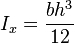# Beam Deflection equation question

• amir azlan

#### amir azlan

regarding this equation,

δ=(PL^3 )/(48EI)

For the calculation of maximum deflection, if my structure is not longer a full form beam (meaning the structure has 6 holes drilled to it), can this formula still be used?is this formula still valid for this structure?

regarding this equation,

δ=(PL^3 )/(48EI)

For the calculation of maximum deflection, if my structure is not longer a full form beam (meaning the structure has 6 holes drilled to it), can this formula still be used?is this formula still valid for this structure?
It depends on where the holes are located in this particular beam.

It depends on where the holes are located in this particular beam.
Attached is the the drawing of the beam. 3 holes drilled to each end of the beam.
How to calculate the max deflection of beam, as the given formula of δ=(PL^3 )/(48EI) is no longer valid?

#### Attachments

Attached is the the drawing of the beam. 3 holes drilled to each end of the beam.
How to calculate the max deflection of beam, as the given formula of δ=(PL^3 )/(48EI) is no longer valid?
The central deflection of a loaded beam also depends on how the ends are supported. The formula given is for calculating the central deflection of a beam with a single load P located in the middle of the span.

It's not clear from the description of the beam presented so far for what purpose the holes are drilled in the end. If these holds are placed there to allow bolts or rivets to attach this beam to another member for support at the ends, then the assumption that this beam is simply supported is probably not valid, in which case the formula for calculating deflection is also not valid.

Please provide more details about how the beam is supported or attached to a larger structure.

yes,these holes are drilled to hold the beam onto the frame using bolts.
In, this case, the beam ends are not free to rotate once bolted to the supporting structural supports, so the deflection formula mentioned in the OP is not valid for calculating the deflection in this case.

The formula for calculating the central deflection of a beam with both ends fixed with a single concentrated load P applied mid-span is ##δ=\frac{PL^3}{192EI} ##. Here, L should be taken as the length of the span between the innermost bolts.

For the moment of inertia,I of the beam,is it still calculated using the formula?

For the moment of inertia,I of the beam,is it still calculated using the formula?
Yes.

From your diagrams, it appears that h < b.

Yes.

From your diagrams, it appears that h < b.
Yes, the dimension of the beam:
30mm width, 5mm thick